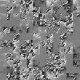author Amos RobinsonWed, 14 Nov 2012 03:38:50 +0000 (14:38 +1100) committer Amos RobinsonWed, 14 Nov 2012 03:38:50 +0000 (14:38 +1100)
 dph-examples/dph-examples.cabal patch | blob | history dph-examples/dph-examples.template patch | blob | history dph-examples/examples/imaginary/StringSearch/Main.hs [new file with mode: 0644] patch | blob dph-examples/examples/imaginary/StringSearch/Vector.hs [new file with mode: 0644] patch | blob dph-examples/examples/imaginary/StringSearch/Vectorised.hs [new file with mode: 0644] patch | blob

index 5849b7b..b05ea24 100644 (file)
@@ -84,6 +84,15 @@ Executable dph-smoke-reverse
-- hs-source-dirs: examples/imaginary/Primes lib
-- ghc-options: -eventlog -rtsopts -threaded -fllvm -optlo-O3 -Odph -package dph-lifted-vseg -fcpr-off -fsimpl-tick-factor=1000

+Executable dph-imaginary-stringsearch
+  Build-depends: base == 4.6.*, vector == 0.9.*, random == 1.0.*, old-time == 1.1.*, containers == 0.5.*, dph-base == 0.8.*, dph-prim-par == 0.8.*, dph-lifted-vseg == 0.8.*, HUnit == 1.2.*, repa-flow == 3.2.*
+  Main-is: Main.hs
+  other-modules: Vectorised
+                  Vector
+                  Timing
+  hs-source-dirs: examples/imaginary/StringSearch lib
+  ghc-options: -eventlog -rtsopts -threaded -fllvm -optlo-O3 -Odph -package dph-lifted-vseg -fcpr-off -fsimpl-tick-factor=1000
+
Executable dph-imaginary-words
Build-depends: base == 4.6.*, vector == 0.9.*, random == 1.0.*, old-time == 1.1.*, containers == 0.5.*, dph-base == 0.8.*, dph-prim-par == 0.8.*, dph-lifted-vseg == 0.8.*, HUnit == 1.2.*, repa-flow == 3.2.*
Main-is: Main.hs
@@ -153,6 +162,16 @@ Executable dph-spectral-quicksort
ghc-options: -eventlog -rtsopts -threaded -fllvm -optlo-O3 -Odph -package dph-lifted-vseg -fcpr-off -fsimpl-tick-factor=1000

+Executable dph-spectral-quickselect
+  Build-depends: base == 4.6.*, vector == 0.9.*, random == 1.0.*, old-time == 1.1.*, containers == 0.5.*, dph-base == 0.8.*, dph-prim-par == 0.8.*, dph-lifted-vseg == 0.8.*, HUnit == 1.2.*, repa-flow == 3.2.*
+  Main-is: Main.hs
+  other-modules: Vectorised
+                  Vector
+                  Timing
+  hs-source-dirs: examples/spectral/QuickSelect/dph lib
+  ghc-options: -eventlog -rtsopts -threaded -fllvm -optlo-O3 -Odph -package dph-lifted-vseg -fcpr-off -fsimpl-tick-factor=1000
+
+
-- Real -----------------------------------------------------------------------
--Executable dph-real-nbody-gloss
-- Main-is: MainGloss.hs
index 70a7de2..6677ce0 100644 (file)
@@ -84,6 +84,15 @@ Executable dph-smoke-reverse
--   hs-source-dirs: examples/imaginary/Primes lib
--   ghc-options:    DPH_OPTIONS

+Executable dph-imaginary-stringsearch
+  Build-depends:  DPH_DEPENDS
+  Main-is:        Main.hs
+  other-modules:  Vectorised
+                  Vector
+                  Timing
+  hs-source-dirs: examples/imaginary/StringSearch lib
+  ghc-options:    DPH_OPTIONS
+
Executable dph-imaginary-words
Build-depends:  DPH_DEPENDS
Main-is:        Main.hs
@@ -153,6 +162,16 @@ Executable dph-spectral-quicksort
ghc-options:    DPH_OPTIONS

+Executable dph-spectral-quickselect
+  Build-depends:  DPH_DEPENDS
+  Main-is:        Main.hs
+  other-modules:  Vectorised
+                  Vector
+                  Timing
+  hs-source-dirs: examples/spectral/QuickSelect/dph lib
+  ghc-options:    DPH_OPTIONS
+
+
-- Real -----------------------------------------------------------------------
--Executable dph-real-nbody-gloss
--    Main-is:        MainGloss.hs
diff --git a/dph-examples/examples/imaginary/StringSearch/Main.hs b/dph-examples/examples/imaginary/StringSearch/Main.hs
new file mode 100644 (file)
index 0000000..8fda7d6
--- /dev/null
@@ -0,0 +1,84 @@
+
+import Vector
+import Vectorised
+import Timing
+import Data.Array.Parallel
+import qualified Data.Array.Parallel.Prelude.Word8    as W
+import qualified Data.Array.Parallel.PArray         as P
+import qualified Data.Array.Parallel.Unlifted        as U
+import Data.Char
+import System.Environment
+import qualified Data.Vector.Unboxed    as VU
+
+str    =  "When   I   look  into  the   looking glass I'm always sure to see"
+       ++ " no matter how I dodge         about, me looking      back at me."
+
+search = "look"
+
+main :: IO ()
+main
+ = do  args    <- getArgs
+       case args of
+         [alg,replCount]
+           -> run alg (read replCount)
+
+         _ -> do
+               putStr usage
+               return ()
+
+
+-- | Command line usage information.
+usage :: String
+usage  = unlines
+       [ "Usage: stringsearch <vector|vectorised> <points>"    ]
+
+
+run "vectorised"  n
+ = do
+    let str' = concat \$ replicate n str
+
+    -- convert string to a PArray
+    let arrOfStr = P.fromUArray . U.map W.fromInt . U.fromList . map ord
+
+    let paStr   :: PArray W.Word8
+        paStr = arrOfStr str'
+
+    let paSearch:: PArray W.Word8
+        paSearch = arrOfStr search
+
+    paStr `seq` paSearch `seq` return ()
+
+    -- find indices of search in string
+    (res, tElapsed)
+        <- time
+        \$  let s = P.toUArray \$ searchPA paSearch paStr
+           in  s `seq` return s
+
+    putStr \$ "results  = " ++ show res ++ "\n"
+    putStr \$ prettyTime tElapsed
+
+run "vector"  n
+ = do
+    let str' = concat \$ replicate n str
+
+    -- convert string to a PArray
+    let arrOfStr = VU.fromList
+
+    let paStr   :: VU.Vector Char
+        paStr = arrOfStr str'
+
+    let paSearch:: VU.Vector Char
+        paSearch = arrOfStr search
+
+    paStr `seq` paSearch `seq` return ()
+
+    -- find indices of search in string
+    (res, tElapsed)
+        <- time
+        \$  let s = searchV paSearch paStr
+           in  s `seq` return s
+
+    putStr \$ "results  = " ++ show res ++ "\n"
+    putStr \$ prettyTime tElapsed
+
+
diff --git a/dph-examples/examples/imaginary/StringSearch/Vector.hs b/dph-examples/examples/imaginary/StringSearch/Vector.hs
new file mode 100644 (file)
index 0000000..6258599
--- /dev/null
@@ -0,0 +1,29 @@
+module Vector
+       ( searchV )
+where
+import qualified Data.Vector            as V
+import qualified Data.Vector.Unboxed    as U
+import Prelude hiding (String)
+
+
+type String    = U.Vector Char
+
+next_character :: U.Vector Int -> String -> String -> Int -> U.Vector Int
+next_character candidates w s i
+ | i == U.length w = candidates
+ | otherwise
+ = let letter      = w U.! i
+       next_l      = U.map (\ix -> s U.! (ix + i)) candidates
+       (candidates',_) = U.unzip (U.filter (\(_,n) -> n == letter) (candidates `U.zip` next_l))
+   in  next_character candidates' w s (i + 1)
+
+string_search :: String -> String -> U.Vector Int
+string_search w s = next_character (U.enumFromN 0 (U.length s - U.length w + 1)) w s 0
+
+-- Interface ------------------------------------------------------------------
+
+-- | SEARCH
+{-# NOINLINE searchV #-}
+searchV :: String -> String -> U.Vector Int
+searchV w s = string_search w s
+
diff --git a/dph-examples/examples/imaginary/StringSearch/Vectorised.hs b/dph-examples/examples/imaginary/StringSearch/Vectorised.hs
new file mode 100644 (file)
index 0000000..1e09b4c
--- /dev/null
@@ -0,0 +1,38 @@
+{-# LANGUAGE ParallelArrays, ParallelListComp #-}
+{-# OPTIONS -fvectorise #-}
+
+module Vectorised
+       ( searchPA )
+where
+import qualified Data.Array.Parallel.Prelude.Word8     as W
+import Data.Array.Parallel.Prelude.Word8               (Word8)
+import Data.Array.Parallel.Prelude.Int                  as I
+import Data.Array.Parallel
+
+import qualified Prelude as Prel
+
+
+-- We can't use the Prelude Char and String types in vectorised code yet..
+type Char      = Word8
+
+type String    = [: Char :]
+
+next_character :: [:Int:] -> String -> String -> Int -> [:Int:]
+next_character candidates w s i
+ | i I.== lengthP w = candidates
+ | otherwise
+ = let letter      = w !: i
+       next_l      = mapP (\ix -> s !: (ix I.+ i)) candidates
+       (candidates',_) = unzipP (filterP (\(_,n) -> n W.== letter) (candidates `zipP` next_l))
+   in  next_character candidates' w s (i I.+ 1)
+
+string_search :: String -> String -> [:Int:]
+string_search w s = next_character (enumFromToP 0 (lengthP s I.- lengthP w I.+ 1)) w s 0
+
+-- Interface ------------------------------------------------------------------
+
+-- | SEARCH
+{-# NOINLINE searchPA #-}
+searchPA :: PArray Word8 -> PArray Word8 -> PArray Int
+searchPA w s = toPArrayP (string_search (fromPArrayP w) (fromPArrayP s))
+